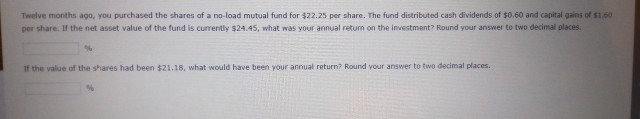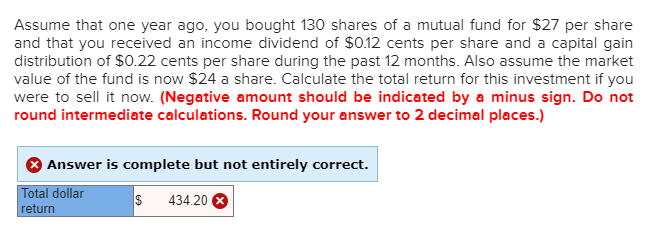# Twelve months ago, you purchased the shares of a no-load mutual fund for \$22.25 per share....Twelve months ago, you purchased the shares of a no-load mutual fund for \$22.25 per share. The fund distributed cash dividends of \$0.60 and capital gains of \$1.60 per share. If the net asset value of the fund is currently \$24.45, what was your annual return on the investment? Round your answer to two decimal places If the value of the shares had been \$21.18, what would have been your annual return? Round your answer to two decimal places.

Annual Return on Investment = (Dividend distribution + Capital gains distribution + Change in net asset value) / Beginning Net Asset Value

Where Change in net asset value = Ending Net Asset Value - Beginning Net Asset Value

Dividend distribution = \$0.60

Capital gains distribution = \$1.60

Beginning Net Asset Value = \$22.25

Ending Net Asset Value = \$24.45

Change in net asset value = \$24.45 - \$22.25

= \$2.20

Therefore Annual Return on Investment =  (\$0.60 + \$1.60 + \$2.20) / \$22.25

= 4.40 / 22.25

= 0.1978 or

19.78 % (Rounded two decimal places)

Dividend distribution = \$0.60

Capital gains distribution = \$1.60

Beginning Net Asset Value = \$22.25

Ending Net Asset Value = \$21.18

Change in net asset value = \$21.18 - \$22.25

= - \$1.07

Therefore Annual Return on Investment =  (\$0.60 + \$1.60 + (- \$1.07) / \$22.25

= 1.13 / 22.25

= 0.0508 or

5.08 % (Rounded two decimal places)

##### Add Answer of: Twelve months ago, you purchased the shares of a no-load mutual fund for \$22.25 per share....
Similar Homework Help Questions
• ### You purchased shares of a mutual fund at an offering price of \$95 per share at...

You purchased shares of a mutual fund at an offering price of \$95 per share at the beginning of the year and paid a front–end load of 4.00%. If the securities in which the fund invested increased in value by 13.25% during the year, and the fund’s expense ratio was 1.50%, what is your rate of return if you sold the fund at the end of the year? Enter your answer rounded to two decimal places. The price of a...

• ### You purchased shares of a mutual fund at a price of \$24.6 per share two years...

You purchased shares of a mutual fund at a price of \$24.6 per share two years ago and paid a front-end load of 4.8% at that point. Assume that the securities in which the fund invested in increased in value by 5.7% during the first year and 4.7% during the second year. Assume further that the fund's expense ratio was 2.4%. Your total return if you sold the fund at the end of the second year would be ______%. Answer...

• ### Assume that one year ago you bought 90 shares of a mutual fund for \$18 per...

Assume that one year ago you bought 90 shares of a mutual fund for \$18 per share, you received a capital gain distribution of \$0.38 per share during the past 12 months, and the market value of the fund is now \$23. Calculate the total return for this investment if you were to sell it now. (Negative amount should be indicated by a minus sign. Do not round intermediate calculations. Round your answer to 2 decimal places.) Total Dollar Return:

• ### If an investor buys shares in a no-load mutual fund for \$32 and after nine years...

If an investor buys shares in a no-load mutual fund for \$32 and after nine years the shares appreciate to \$57, what is (1) the percentage return and (2) the annual compound rate of return using time value of money? Round your answers to two decimal places.

• ### Assume that one year ago, you bought 130 shares of a mutual fund for \$27 per...Assume that one year ago, you bought 130 shares of a mutual fund for \$27 per share and that you received an income dividend of \$0.12 cents per share and a capital gain distribution of \$0.22 cents per share during the past 12 months. Also assume the market value of the fund is now \$24 a share. Calculate the total return for this investment if you were to sell it now. (Negative amount should be indicated by a minus sign....

• ### If an investor buys shares in a no-load mutual fund for \$33 and after five years...

If an investor buys shares in a no-load mutual fund for \$33 and after five years the shares appreciate to \$57, what is (1) the percentage return and (2) the annual compound rate of return using time value of money? Round your answers to two decimal places. Percentage return: 0.7273 % The annual compound rate of return: %

• ### You purchased shares of a mutual fund at an offering price of \$95 per share at...

You purchased shares of a mutual fund at an offering price of \$95 per share at the beginning of the year and paid a front-end load of 4.25%. If the securities in which the fund invested increased in value by 9.75% during the year, and the fund's expense ratio was 1.90%, what is your rate of return if you sold the fund at the end of the year?

• ### You purchased shares of a mutual fund at a price of \$27 per share at the...

You purchased shares of a mutual fund at a price of \$27 per share at the beginning of the year and paid a front-end load of 1.2%. If the securities in which the fund invested increased in value by 12.9% during the year, and the fund's expense ratio was 2.6%, your return if you sold the fund at the end of the year would be ____________%. Select one: a. 12.9 b. -3.8 c. 11.5 d. 10.3 e. 9.0

• ### Suppose an individual invests \$40,000 in a load mutual fund for two years. The load fee...

Suppose an individual invests \$40,000 in a load mutual fund for two years. The load fee entails an up-front commission charge of 3 percent of the amount invested and is deducted from the original funds invested. In addition, annual fund operating expenses (or 12b-1 fees) are 0.60 percent. The annual fees are charged on the average net asset value invested in the fund and are recorded at the end of each year. Investments in the fund return 5 percent each...

• ### Hunter invested ​\$4 comma 000 in shares of a load mutual fund. The load of the...

Hunter invested ​\$4 comma 000 in shares of a load mutual fund. The load of the fund is 5 ​%. When Hunter purchased the​ shares, the NAV per share was ​\$42 . A year​ later, Hunter sold the shares at a NAV of ​\$41 per share. What is​ Hunter's return from selling his shares in the mutual​ fund? ​Hunter's return from selling his shares in the mutual fund is

Need Online Homework Help?